Function Repository Resource:

# Disk3D

Create a BSplineSurface primitive representing a disk

Contributed by: Carl Woll
 ResourceFunction["Disk3D"][{x, y, z}, r] returns a BSplineSurface representation of a 3D disk with center {x,y,z} and radius r, with the normal axis in the z-direction. ResourceFunction["Disk3D"][{x, y, z}, {r1, r2}] returns a 3D elliptical disk with semimajor axis r1 and semiminor axis r2, and with the semimajor axis in the x-direction. ResourceFunction["Disk3D"][{x, y, z},rads, a] returns a 3D elliptical disk with the normal axis in the direction of vector a. ResourceFunction["Disk3D"][{x, y, z}, rads, {u, v}] returns a 3D elliptical disk with the semimajor axis in the direction u, and with the ellipical plane spanned by vectors u and v.

## Details and Options

ResourceFunction["Disk3D"] can be used in Graphics3D.
ResourceFunction["Disk3D"] returns a BSplineSurface object.
ResourceFunction["Disk3D"][] is equivalent to ResourceFunction["Disk3D"][{0,0,0},1,{{1,0,0},{0,1,0}}].
Graphics rendering is affected by directives such as FaceForm, EdgeForm and color.

## Examples

### Basic Examples (3)

A 3D disk:

 In:=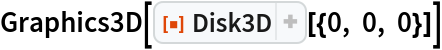Out=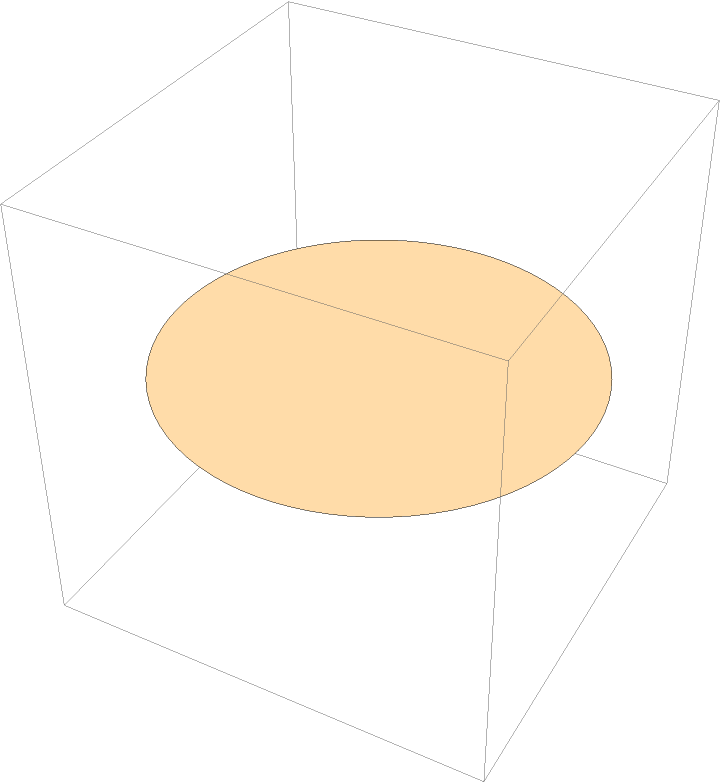A 3D elliptical disk:

 In:=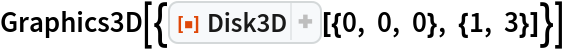Out=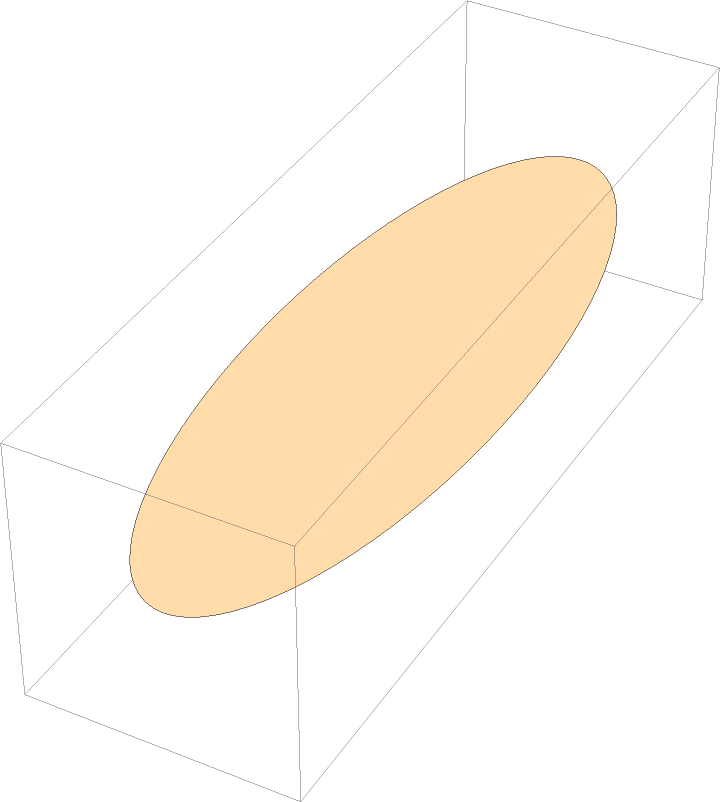Differently-styled disks:

 In:=Out=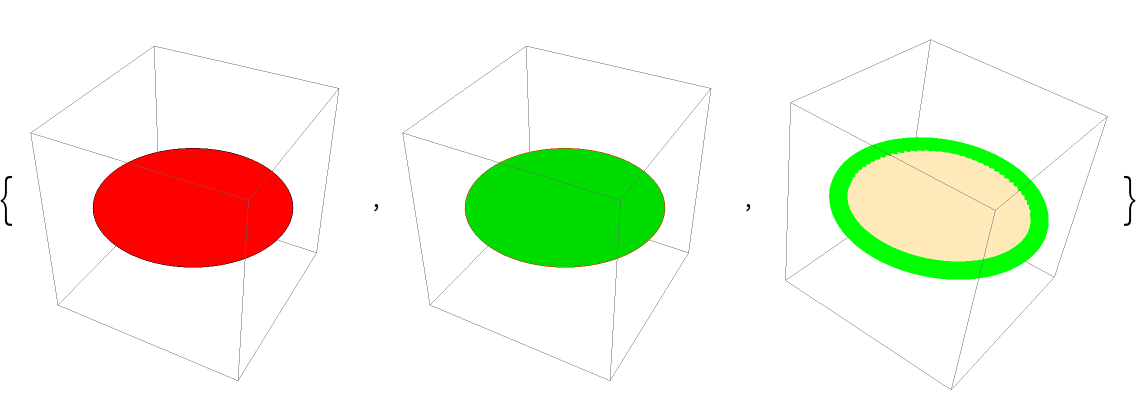### Scope (3)

A disk with a normal in the direction {1,1,1}:

 In:=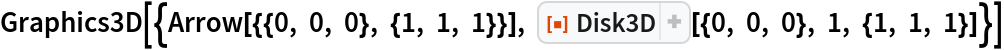Out=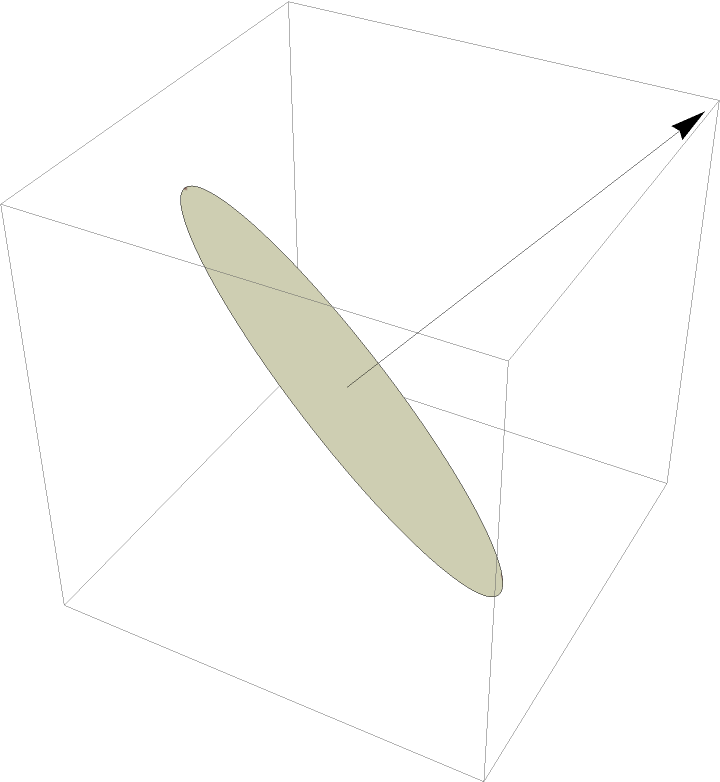A disk in the plane spanned by the vectors {1,1,0} and {0,1,1}:

 In:=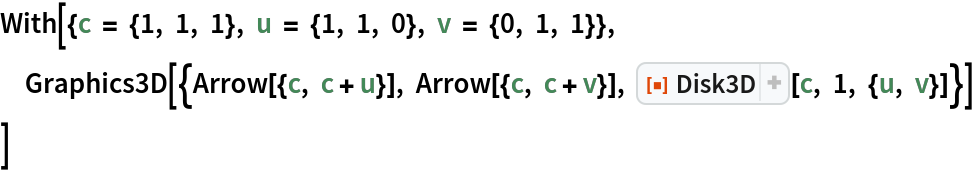Out=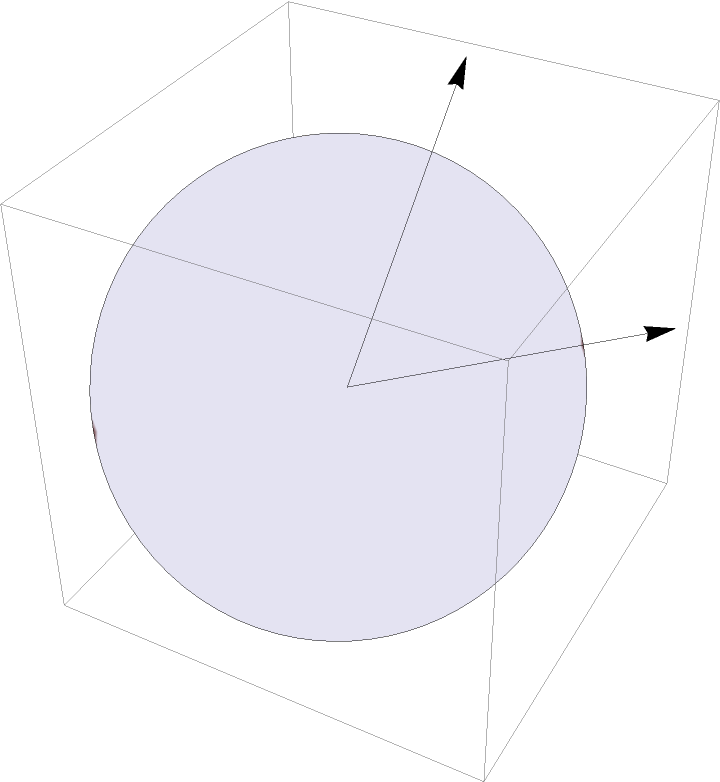Rendering of a fairly large number of disks:

 In:=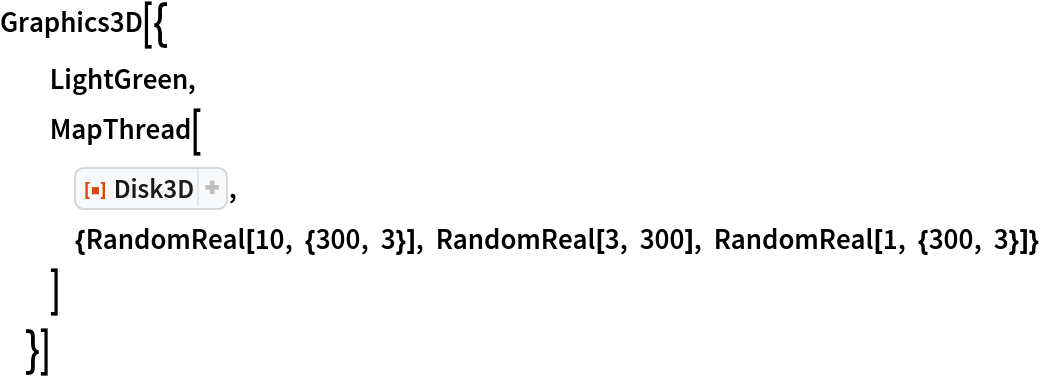Out=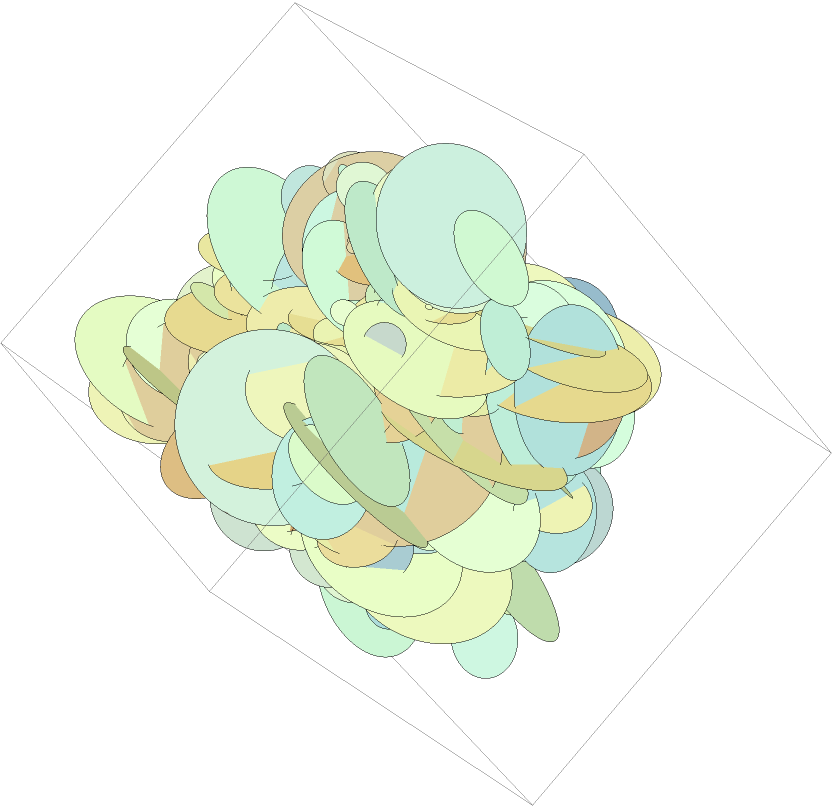Carl Woll

## Version History

• 1.0.0 – 16 December 2019